Civil protection

Students on civil protection exercise went 5800 m long trip. How many kilometers is it approximately?

Result

x =  6 km

Solution:Leave us a comment of example and its solution (i.e. if it is still somewhat unclear...):Be the first to comment!To solve this example are needed these knowledge from mathematics:

Do you want to convert length units?

Next similar examples:

1. Math classificationIn 3A class are 27 students. One-third got a B in math and the rest got A. How many students received a B in math?
2. Bean bag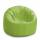A student tossed a bean bag. It landed 216 inches away. How many yards are equal to 216 inches?
3. Jumps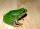At least how many jumps must do frog to overcome the distance of 7 meters? Jump of frog is 18 cm long.
4. StepsWalkway from the house to the school is 180 m long. How many more steps Michael makes than his father on the way to school, if the length of Michael's step is 60 cm and the length of his father's step is 90 cm.
5. Wire cut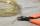A wire of length 7 m was cut into equal lengths using 4 cuts. How long is each piece?
6. Shaving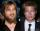Mathematical problem from Sunday evening. If the beard is growing at 0.52 mm per day and that my length is 16.8 mm, how long I don't shave?
7. The resultHow many times I decrease the number 1632 to get the result 24?
8. Crates 2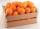One crate will hold 50 oranges. If Bob needs to ship 932 oranges, how many crates will he need?
9. DoctorsIn the city operates 171 doctors. The city has 128934 citizens. How many citizens are per one doctor?
10. Steps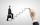Adult step has a length 76 cm. How many steps will he goes distance 50 meters? How many meters he goes when he makes 700 steps?
11. Like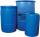When is in 14 barrels 140 liters of water, how many liters barrels of 8 liters I need to get all the water from the larger barrels?
12. To thousands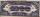Round to thousands following numbers:
13. Valid numberRound the 453874528 on 2 significant numbers.Added together and write as decimal number: LXVII + MLXIV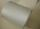Salesman account for 2.3 meters of wallpaper 149.50 Kc. How much cost 1 meter of wallpaper?Children break chocolate first to third and then every part of another half. What kind got each child? Draw a picture. What part would have received if each piece have halved?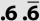Double round number 727, first to tens, then to hundreds. (double rounding)